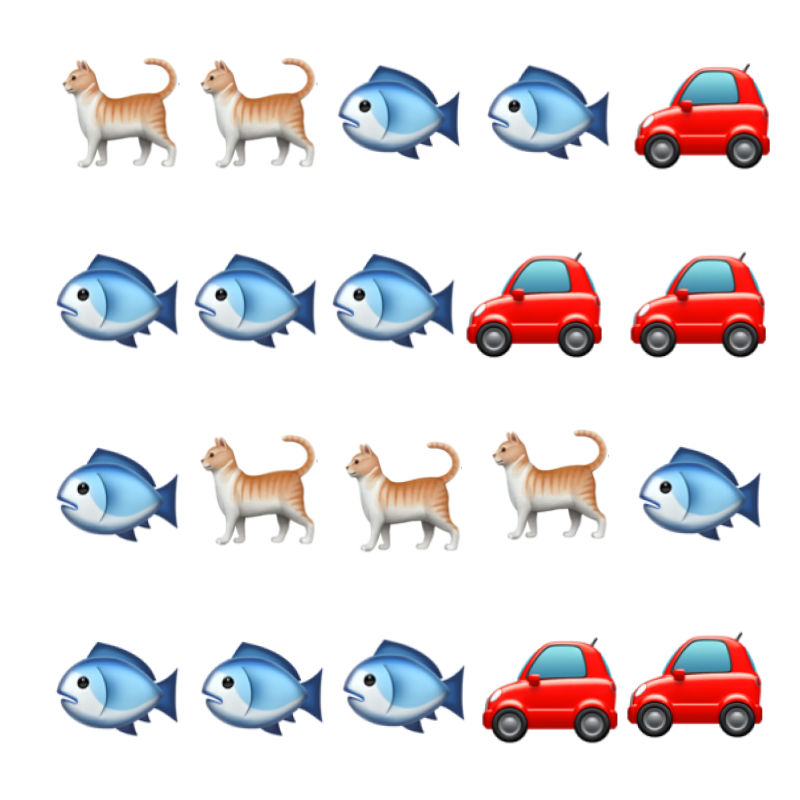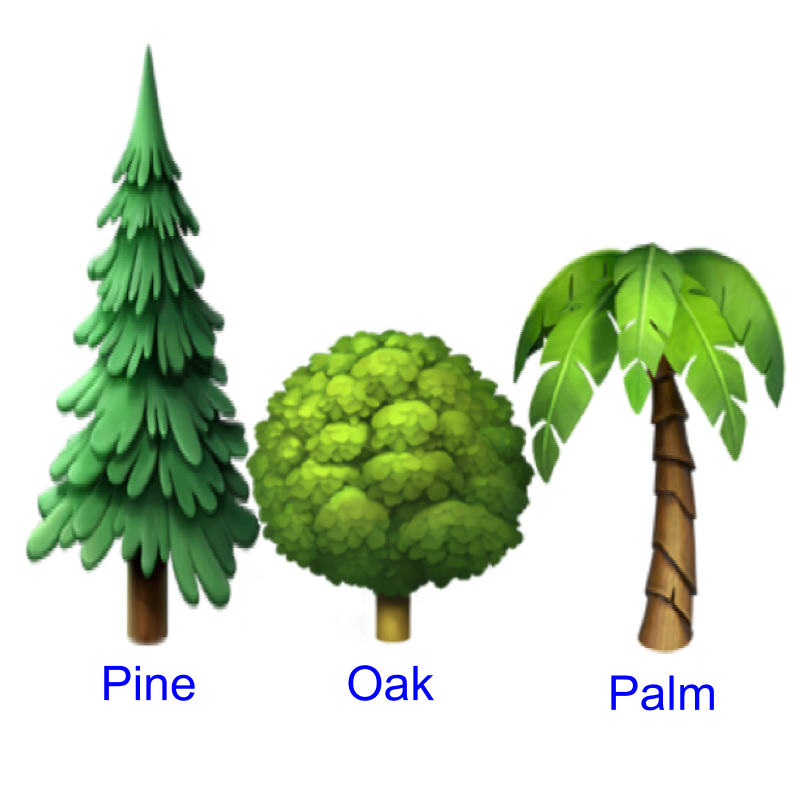maths > wholenumbers

Largest or Smallest (Whole Numbers)

what you'll learn...

overview

To find largest or smallest number among three or more numbers, a simplified procedure or algorithm is explained.

many numbers & one largestConsider the number of cats, fish, and cars in the figure. The number of fish is the largest among the given three numbers.

Count of Quantities can be compared to find the largest or smallest among them.Consider the three trees shown in the figure. The pine tree, oak tree, and the palm tree. The pine tree is the tallest in the given three trees.

Measurement of Quantities such as height, weight, etc. can be compared to find the largest or smallest among them.

Find the largest in the following 234$234$, 23$23$, 423$423$.
The answer is "423$423$"

•  More than two numbers can be compared to find the largest or smallest among them.

Simplified procedure to find Largest (or Smallest) among many numbers : Compare the first number with the second one. Take the largest (or smallest) and compare that with the next number. Taking the largest (or smallest) in every step, compare with the next number till all the numbers are compared. The largest (or smallest) in the last step is the result.

examples

Find the largest in the following 78$78$, 22$22$, $322$.
The answer is "$322$"

Find the smallest in the following $78$, $22$, $322$.
The answer is "$22$"

Find the largest in the following $2$, $2$, $2$.
The answer is "$2$". Largest among them is the value $2$.

summary

Simplified procedure to find Largest (or Smallest) among many numbers : Compare the first number with the second one. Take the largest (or smallest) and compare that with the next number. Taking the largest (or smallest) in every step, compare with the next number till all the numbers are compared. The largest (or smallest) in the last step is the result.

Outline